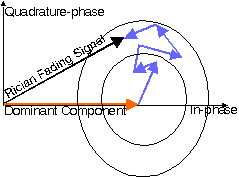JPL's Wireless Communication Reference Website

Chapter: Wireless Channels Section: Multipath FadingThe model behind Rician fading is similar to that for Rayleigh fading, except that in Rician fading a strong dominant component is present. This dominant component can for instance be the line-of-sight wave. Refined Rician models also consider that
• that the dominant wave can be a phasor sum of two or more dominant signals, e.g. the line-of-sight, plus a ground reflection. This combined signal is then mostly treated as a deterministic (fully predictable) process, and that
• the dominant wave can also be subject to shadow attenuation. This is a popular assumption in the modelling of satellite channels.
Besides the dominant component, the mobile antenna receives a large number of reflected and scattered waves.Phasor Diagram of Rician fading signal Java Applet: Phasor diagram of one direct and 4 scattered waves (in blue), resulting an approximately Rice-fading envelope (in black).

Signal ModelHear a discussion of the basic model behind Ricain and Rayleigh fading.

We model a narrowband propagation channel by considering a sinusoidal transmitted carrier

s(t) = cos wct

This signal received over a Rician multipath channel can be expressed as

v(t) = C cos wct + SNn=1   rn cos (wct + fn)
where
C is the amplitude of the line-of-sight component
rn is the amplitude of the n-th reflected wave
fn is the phase of the n-th reflected wave
n = 1 .. N identify the reflected, scattered waves.
Rayleigh fading is recovered for C= 0

PDF of signal amplitude

The derivation of the probability density function of amplitude is more involved than for Rayleigh fading, and a Bessel function occurs in the mathematical expression. It has been proposed to approximate this expression by the model for Nakagami fading, however, the behaviour of Nakagami and Rician fading in deep fades is essentially different. Approximations that focus on the behavior near the mean value, will divert by orders of magnitude in predicting the probability of deep fades.

Rician factor

The Rician K-factor is defined as the ratio of signal power in dominant component over the (local-mean) scattered power. In the expression for the received signal, the power in the line-of-sight equals C2/2. In indoor channels with an unobstructed line-of-sight between transmit and receive antenna the K-factor is between, say, 4 and 12 dB. Rayleigh fading is recovered for K = 0 (-infinity dB).